# Python多进程和多线程（跑满CPU）

https://www.liaoxuefeng.com/wiki/1016959663602400/1017627212385376

Python多进程和多线程（跑满CPU

• 概念

• 多进程模式；

• 多线程模式

• 多进程+多线程模式。

• 多进程

multiprocessing模块提供了一个Process类来代表一个进程对象。

multiprocessing模块提供了一个Pool进程池的方式批量创建子进程。

from multiprocessing import Pool

import os, time

print('Run task %s (%s)...' % (name, os.getpid()))

start = time.time()

time.sleep(1)

end = time.time()

print('Task %s runs %0.2f seconds.' % (name, (end - start)))

if __name__=='__main__':

print('Parent process %s.' % os.getpid())

p = Pool(5)

for i in range(10):

print('Waiting for all subprocesses done...')

p.close()

p.join()

print('All subprocesses done.')

Parent process 13780.

Waiting for all subprocesses done...

All subprocesses done.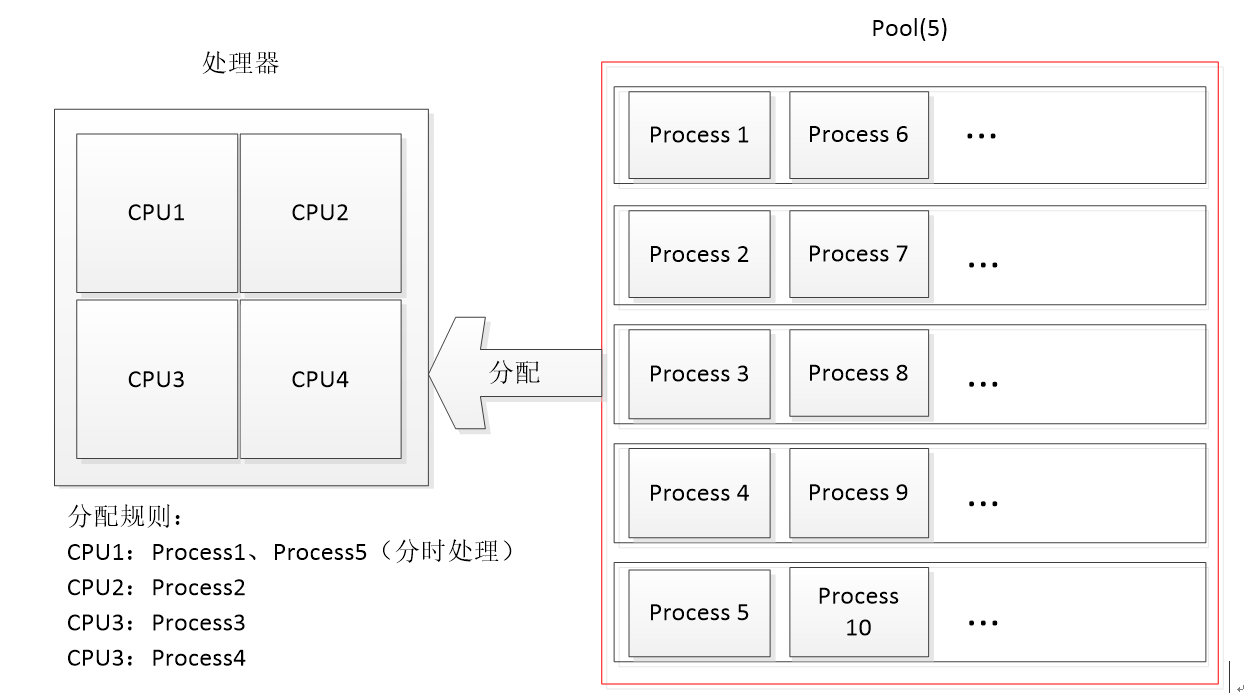p = Pool(10)就可以同时跑10个进程。

• 子进程

• 进程间的通信

Process之间肯定是需要通信的，操作系统提供了很多机制来实现进程间的通信。Pythonmultiprocessing模块包装了底层的机制，提供了QueuePipes等多种方式来交换数据。

from multiprocessing import Process, Queue

import os, time, random

# 写数据进程执行的代码:

def write(q):

print('Process to write: %s' % os.getpid())

for value in ['A', 'B', 'C']:

print('Put %s to queue...' % value)

q.put(value)

time.sleep(random.random())

# 读数据进程执行的代码:

print('Process to read: %s' % os.getpid())

while True:

value = q.get(True)

print('Get %s from queue.' % value)

if __name__=='__main__':

# 父进程创建Queue，并传给各个子进程：

q = Queue()

pw = Process(target=write, args=(q,))

# 启动子进程pw，写入:

pw.start()

# 启动子进程pr，读取:

pr.start()

# 等待pw结束:

pw.join()

# pr进程里是死循环，无法等待其结束，只能强行终止:

pr.terminate()

Process to write: 15728

Put A to queue...

Get A from queue.

Put B to queue...

Get B from queue.

Put C to queue...

Get C from queue.

Pipes如何使用？

• 多线程

import time, threading

# 新线程执行的代码:

def loop():

n = 0

while n < 5:

n = n + 1

time.sleep(1)

t.start()

t.join()

print('thread %s ended.' % threading.current_thread().name)

thread MainThread is running...

thread MainThread ended.

Lock：

import time, threading

# 假定这是你的银行存款:

balance = 0

def change_it(n):

# 先存后取，结果应该为0:

global balance

balance = balance + n

balance = balance - n

for i in range(100000):

change_it(n)

t1.start()

t2.start()

t1.join()

t2.join()

print(balance)

Balance = Balance + n

1. 计算临时变量temp = Balance + n
2. 将临时变量temp赋值给Balance

t1: x1 = balance + 5  # x1 = 0 + 5 = 5

t2: x2 = balance + 8  # x2 = 0 + 8 = 8

t2: balance = x2      # balance = 8

t1: balance = x1      # balance = 5

t1: x1 = balance - 5  # x1 = 5 - 5 = 0

t1: balance = x1      # balance = 0

t2: x2 = balance - 8  # x2 = 0 - 8 = -8

t2: balance = x2   # balance = -8

balance = 0

for i in range(100000):

# 先要获取锁:

lock.acquire()

try:

# 放心地改吧:

change_it(n)

finally:

# 改完了一定要释放锁:

lock.release()

• 多核CPU

import threading, multiprocessing

def loop():

x = 0

while True:

x = x ^ 1

for i in range(multiprocessing.cpu_count()):

t.start()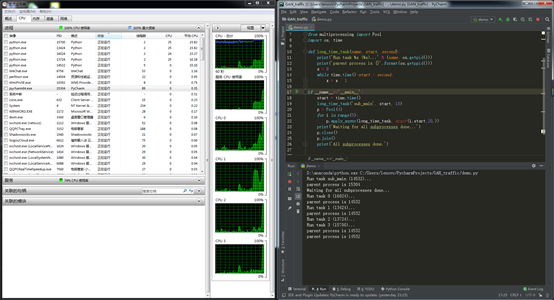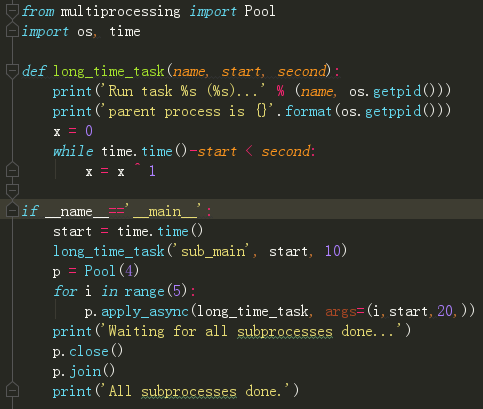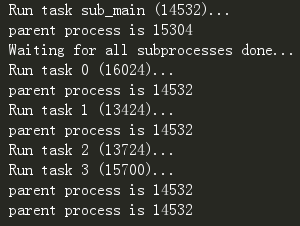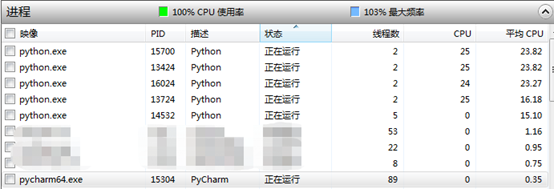• 进程VS.线程

IO密集型任务执行期间，99%的时间都花在IO上，花在CPU上的时间很少，因此，用运行速度极快的C语言替换用Python这样运行速度极低的脚本语言，完全无法提升运行效率。对于IO密集型任务，最合适的语言就是开发效率最高（代码量最少）的语言，脚本语言是首选，C语言最差。

• 小结

• 分布式进程

ThreadProcess中，应当优选Process，因为Process更稳定，而且，Process可以分布到多台机器上，而Thread最多只能分布到同一台机器的多个CPU上。

Python的multiprocessing模块不但支持多进程，其中managers子模块还支持把多进程分布到多台机器上。一个服务进程可以作为调度者，将任务分布到其他多个进程中，依靠网络通信。由于managers模块封装很好，不必了解网络通信的细节，就可以很容易地编写分布式多进程程序。

# task_master.py

import random, time, queue

from multiprocessing.managers import BaseManager

# 发送任务的队列:

# 接收结果的队列:

result_queue = queue.Queue()

# 从BaseManager继承的QueueManager:

class QueueManager(BaseManager):

pass

# 把两个Queue都注册到网络上, callable参数关联了Queue对象:

QueueManager.register('get_result_queue', callable=lambda: result_queue)

# 绑定端口5000, 设置验证码'abc':

# 启动Queue:

manager.start()

# 获得通过网络访问的Queue对象:

result = manager.get_result_queue()

# 放几个任务进去:

for i in range(10):

n = random.randint(0, 10000)

# 从result队列读取结果:

print('Try get results...')

for i in range(10):

r = result.get(timeout=10)

print('Result: %s' % r)

# 关闭:

manager.shutdown()

print('master exit.')



# task_worker.py

import time, sys, queue

from multiprocessing.managers import BaseManager

# 创建类似的QueueManager:

class QueueManager(BaseManager):

pass

# 由于这个QueueManager只从网络上获取Queue，所以注册时只提供名字:

QueueManager.register('get_result_queue')

print('Connect to server %s...' % server_addr)

# 从网络连接:

m.connect()

# 获取Queue的对象:

result = m.get_result_queue()

for i in range(10):

try:

print('run task %d * %d...' % (n, n))

r = '%d * %d = %d' % (n, n, n*n)

time.sleep(1)

result.put(r)

except Queue.Empty:

# 处理结束:

print('worker exit.')

$python3 task_master.py Put task 3411... Put task 1605... Put task 1398... Put task 4729... Put task 5300... Put task 7471... Put task 68... Put task 4219... Put task 339... Put task 7866... Try get results... task_master.py进程发送完任务后，开始等待result队列的结果。现在启动task_worker.py进程：$ python3 task_worker.py

Connect to server 127.0.0.1...

worker exit.

task_worker.py进程结束，在task_master.py进程中会继续打印出结果：

Result: 3411 * 3411 = 11634921

Result: 1605 * 1605 = 2576025

Result: 1398 * 1398 = 1954404

Result: 4729 * 4729 = 22363441

Result: 5300 * 5300 = 28090000

Result: 7471 * 7471 = 55815841

Result: 68 * 68 = 4624

Result: 4219 * 4219 = 17799961

Result: 339 * 339 = 114921

Result: 7866 * 7866 = 61873956

Queue对象存储在哪？注意到task_worker.py中根本没有创建Queue的代码，所以，Queue对象存储在task_master.py进程中：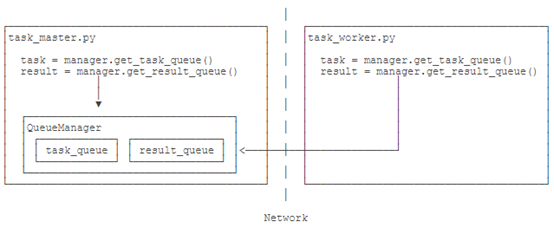Queue之所以能通过网络访问，就是通过QueueManager实现的。由于QueueManager管理的不止一个Queue，所以，要给每个Queue的网络调用接口起个名字，比如get_task_queue

authkey有什么用？这是为了保证两台机器正常通信，不被其他机器恶意干扰。如果task_worker.pyauthkeytask_master.pyauthkey不一致，肯定连接不上。

Python的分布式进程接口简单，封装良好，适合需要把繁重任务分布到多台机器的环境下。

03-162804
12-13484
03-236379
11-2151
07-09178
10-14834
09-071643
08-281260
09-16354
11-30104
04-07610
06-08698
11-1491
05-13405
12-06932
10-104345
04-12338
12-04916
10-162万+

### “相关推荐”对你有帮助么？

•非常没帮助
•没帮助
•一般
•有帮助
•非常有帮助点击重新获取扫码支付余额充值